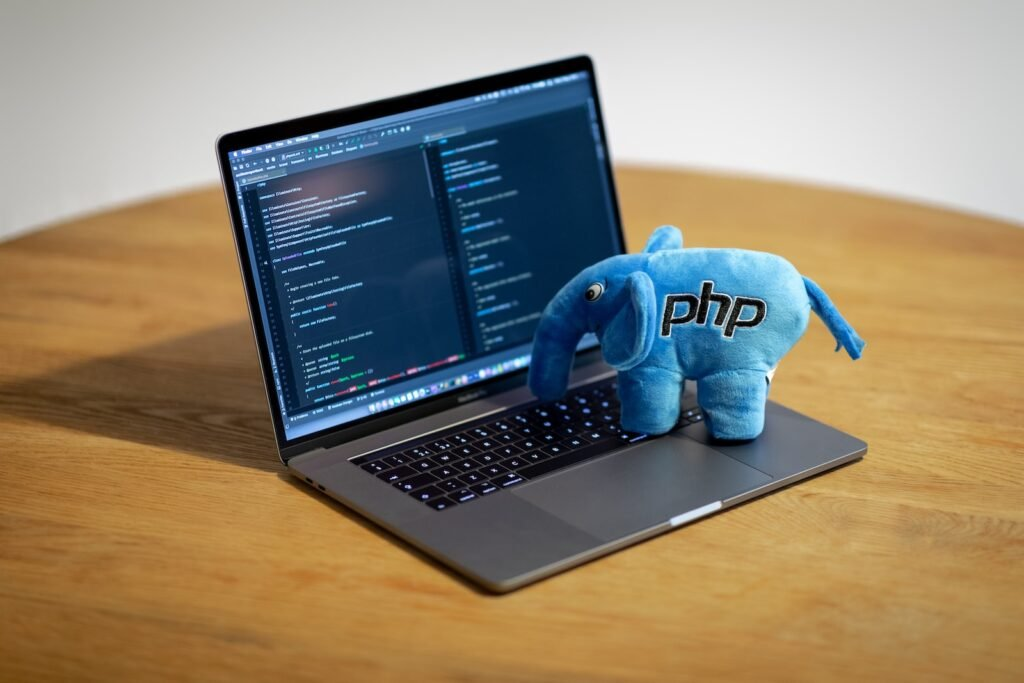## Top 15 php interview questions and answer for fresher candidates1. What is PHP and what is it used for?
PHP is a server-side scripting language used to develop web applications. It can be embedded into HTML and is commonly used to create dynamic websites, manage databases, and perform other server-side tasks.
2. What is a PHP script?
A PHP script is a series of instructions written in the PHP language that is executed on the server when a PHP page is accessed by a user.
3. How is PHP different from other programming languages?
PHP is a server-side language, which means that it runs on the server and generates HTML that is then sent to the client. This is in contrast to client-side languages such as JavaScript, which run in the user’s web browser.
4. How do you declare a variable in PHP?
In PHP, variables are denoted by a dollar sign (\$) followed by the name of the variable. For example:

\$myVariable = “Hello World”;

1. What are the rules for naming a PHP variable?
• A variable name must start with a letter or an underscore.
• A variable name can only contain alpha-numeric characters and underscores (A-z, 0-9, and _).
• A variable name should not contain spaces or special characters.
1. How do you assign a value to a variable in PHP?
To assign a value to a variable in PHP, you can use the assignment operator (=). For example:

\$myVariable = “Hello World”;

1. What are the different data types in PHP?
PHP supports eight primitive data types:
• integer
• float
• string
• boolean
• array
• object
• null
• resource
1. How do you create a constant in PHP?
To create a constant in PHP, you can use the define() function. For example:

define(“MY_CONSTANT”, “Hello World”);

1. What is an array in PHP?
An array in PHP is a data structure that stores a collection of values, which can be of different types. Arrays are typically used to store lists of items, such as a list of names or a list of products.
2. How do you create an array in PHP?
There are several ways to create an array in PHP. Here are a few examples:

\$myArray = array(“red”, “green”, “blue”);

\$myArray = [“red”, “green”, “blue”];

1. What is a loop in PHP?
A loop is a control structure in PHP that allows you to repeat a block of code a specified number of times. PHP supports several types of loops, including for, while, and do-while loops.
2. What is an if statement in PHP?
An if statement is a control structure in PHP that allows you to execute a block of code if a certain condition is true. If the condition is false, you can specify an optional else block to execute.
3. What is a switch statement in PHP?
A switch statement is a control structure in PHP that allows you to execute a block of code based on the value of a variable. It is similar to an if-else statement, but it can be more efficient in certain cases.
4. What is a function in PHP?
A function is a block of code that performs a specific task and can be reused throughout your PHP code. Functions can accept parameters and return values.
5. How do you create a function in PHP?

To create a function in PHP, you can use the following syntax:

function functionName(\$parameters) { // code to be executed }

Here’s an example of a function that calculates the area of a rectangle:

function calculateArea(\$width, \$height) { \$area = \$width * \$height; return \$area; }

To call the function, you would use the function name followed by a set of parentheses and any required parameters. For example:

\$result = calculateArea(10, 20);

This would call the calculateArea() function with the parameters 10 and 20, and the result would be stored in the \$result variable.